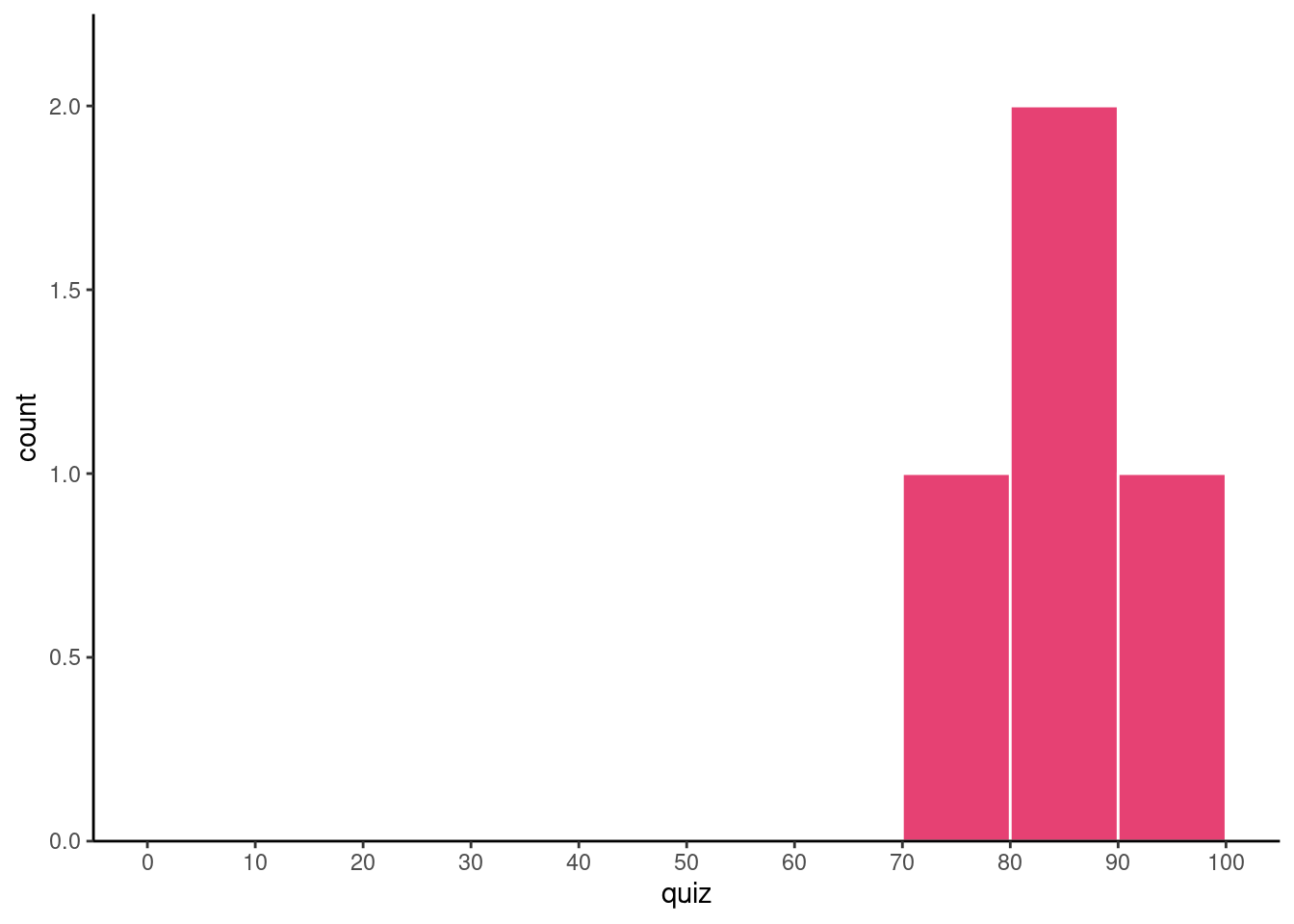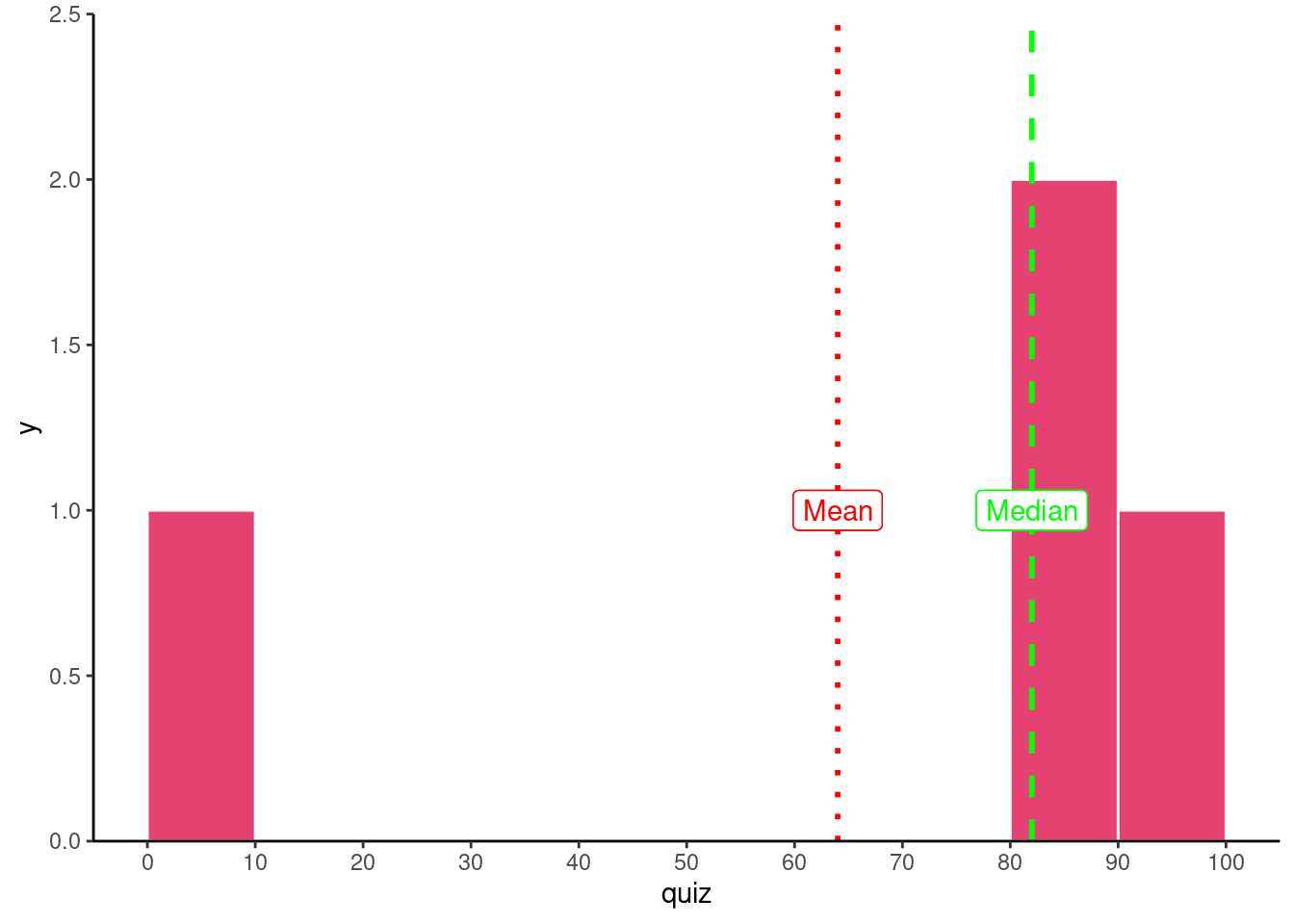# Problem Set 2

Author

Published

September 28, 2022

# Theory and Concepts

## Question 1

In your own words, explain the difference between endogeneity and exogeneity.

An exogenous model is one where the independent variable $$(X)$$ is not associated with any other factors that affect the dependent variable $(Y)$. If a model is truly exogenous, we can estimate the **causal effect** of $$X$$ on $$Y$$.

An endogenous model is one where the independent variable $$(X)$$ is associated with any other factors that affect the dependent variable $$(Y)$$. If a model is endogenous, we have no accurately estimated the causal effect of $$X$$ on $$Y$$, since other factors are getting entangled with $$X$$ and $$Y$$.

## Question 2

### Part A

In your own words, explain what (sample) standard deviation means.

Sample standard deviation measures the average deviation (distance) of any given value of a variable from the variable’s mean.

### Part B

In your own words, explain how (sample) standard deviation is calculated. You may also write the formula, but it is not necessary.

The formula is $sd(X)=\sqrt{\frac\sum^n_{i=1} (x_i-\bar{X})^2}{n-1}$

It helps to consider the calculation as a series of steps:

1. Find the mean of $$X$$, $$(\bar{X})$$
2. Subtract the mean from each value of $$X$$ in the data, to get deviations, $$(x_i-\bar{X})$$
3. Square the deviations to ensure they are all positive, $$(x_i-\bar{X})^2$$
4. Take the average of the squared deviations: add them all up and divide by $$n-1$$, $$\frac{1}{n-1}\displaystyle \sum^n_{i=1} (x_i-\bar{X})^2$$
5. What you have in step 4 is variance (measured in units of $$X^2)$$, square root to get standard deviation (measured in original units of $$X$$): $$\sqrt{\frac{1}{n-1}\displaystyle \sum^n_{i=1} (x_i-\bar{X})^2}$$

Note on Step 4: because this is a sample, we have do deal with degrees of freedom (df) loss. We use up one df to calculate the mean, $$\bar{x}$$, which is needed before calculating variance or standard deviation. Hence, instead of averaging like normal: $$\frac{1}{n} \sum x_i$$, we need to divide by $$n-1$$.

# Problems

For the remaining questions, you may use R to verify, but please calculate all sample statistics by hand and show all work.

## Question 3

Suppose you have a very small class of four students that all take a quiz. Their scores are reported as follows:

$\{83, 92, 72, 81 \}$

### Part A

Calculate the median.

Arrange the values in numerical order from smallest to largest. Find the value in the middle (i.e. an equal number of values are on either side); possibly by crossing-out one number on either side at a time (like in Elementary School).

$\underline{72}, 81, 83, \underline{92}$

Since we have an even number of observations, we have two numbers in the middle, $$81$$ and $$83$$, so we must take the average of them:

$\frac{81+83}{2}=82$

Using R:

median(c(83,92,72,81))
 82

### Part B

Calculate the sample mean, $$\bar{x}$$.

\begin{align*}\bar{x}&=\frac{1}{n} \sum^n_{i=1} x_i\\ \bar{x}&=\frac{72+81+83+92}{4}\\ \bar{x}&=\frac{328}{4}\\ \bar{x}&=82\\ \end{align*}

Using R:

mean(c(83,92,72,81))
 82

### Part C

Calculate the sample standard deviation, $$s$$.

My suggestion is to use the “table” method, and follow the 5 steps described in problem 2b.

$$x_i$$ $$x_i-\bar{X}$$ $$(x_i-\bar{X})^2$$
$$72$$ $$-10$$ $$100$$
$$81$$ $$-1$$ $$1$$
$$83$$ $$1$$ $$1$$
$$92$$ $$10$$ $$100$$
$$\sum$$ $$202$$
$$\frac{1}{3} \times \sum$$ $$\approx 67.33$$
$$\mathbf{\sqrt{\frac{1}{3} \times \sum}}$$ $$\approx \mathbf{8.21}$$

In R:

sd(c(83,92,72,81))
 8.205689

### Part D

Make or sketch a rough histogram of this data, with the size of each bin being 10 (i.e. 70’s, 80’s, 90’s, 100’s). You can draw this by hand or use R.

If you are using ggplot, you want to use + geom_histogram(breaks = seq(start,end,by)) and add another layer + scale_x_continuous(breaks=seq(start,end,by)) . The first layer creates bins in the histogram, and the second layer creates ticks on the x axis; both by creating a sequence starting at some starting value, some ending value, by a certain interval (e.g. by 2, or by 10).

Is this distribution roughly symmetric or skewed? What would we expect about the mean and the median?

# write your code here!
# load tidyverse (for tibble and ggplot2)
library(tidyverse)
── Attaching packages ─────────────────────────────────────── tidyverse 1.3.2 ──
✔ ggplot2 3.3.6      ✔ purrr   0.3.4
✔ tibble  3.1.8      ✔ dplyr   1.0.10
✔ tidyr   1.2.1      ✔ stringr 1.4.1
✔ readr   2.1.2      ✔ forcats 0.5.2
── Conflicts ────────────────────────────────────────── tidyverse_conflicts() ──
✖ dplyr::lag()    masks stats::lag()
# make a dataframe of our data,
# called df
# one variable in it, called quiz

df <- tibble(quiz = c(83,92,72,81))

# use this as our data for plot
ggplot(data = df)+
aes(x = quiz)+
geom_histogram(breaks = seq(0,100,10), # make bins of size 10 between 0 and 100
color = "white", # color is for borders
fill = "#e64173")+ # fill is for area
scale_x_continuous(breaks = seq(0,100,10))+ # have x axix ticks same as breaks
scale_y_continuous(limits = c(0,2.25),
expand = c(0,0))+
theme_classic()### Part E

Suppose instead the person who got the 72 did not show up that day to class, and got a 0 instead. Recalculate the mean and median. What happened and why?

$\underline{0}, 81, 83, \underline{92}$

$\frac{81+83}{2}=82$

Replacing the 72 with a 0, and keeping the same number of observations does not change the median!

\begin{align*}\bar{x}&=\frac{1}{n} \sum^n_{i=1} x_i\\ \bar{x}&=\frac{0+81+83+92}{4}\\ \bar{x}&=\frac{256}{4}\\ \bar{x}&=64\\ \end{align*}

The mean is pulled down significantly by the outlier.

In R:

mean(c(83,92,0,81))
 64
median(c(83,92,0,81))
 82

If we were to look at the histogram, it would be skewed, and the mean would be lower than the mean:

# make new tibble called df_2
df_2 <- tibble(quiz = c(83,92,0,81)) # replace 72 with 0

# use this as our data for plot
ggplot(data = df_2)+
aes(x = quiz)+
geom_histogram(breaks = seq(0,100,10), # make bins of size 10 between 0 and 100
color = "white", # color is for borders
fill = "#e64173")+ # fill is for area
geom_vline(aes(xintercept = median(quiz)),
size = 1,
color = "green",
linetype = "dashed")+ # green dashed line is median
geom_label(aes(x = median(quiz), y = 1),
label = "Median",
color = "green")+ # label median line
geom_vline(aes(xintercept = mean(quiz)),
size = 1,
color = "red",
linetype = "dotted")+ # red dashed line is mean
geom_label(aes(x = mean(quiz), y = 1),
label = "Mean",
color = "red")+ # label mean line
scale_x_continuous(breaks = seq(0,100,10))+ # have x axix ticks same as breaks
scale_y_continuous(limits = c(0,2.5),
expand = c(0,0))+
theme_classic()## Question 4

Suppose the probabilities of a visitor to Amazon’s website buying 0, 1, or 2 books are 0.2, 0.4, and 0.4 respectively.

### Part A

Calculate the expected number of books a visitor will purchase.

$$x_i$$ $$P(X=x_i)$$
0 0.20
1 0.40
2 0.40

The expected value of $$X$$ is the probability weighted average of $$X$$:

\begin{align*}E(X)&=\sum^n_{i=1} p_ix_i\\ &=0.2(0)+(0.4)1+(0.4)2\\ &=0+0.4+0.8\\ &=1.2\\ \end{align*}

### Part B

Calculate the standard deviation of book purchases.

The formula(s) for standard deviation of a random variable is:

$\sigma_X = sd(X) = \sqrt{E[(X-E[X])^2]} = \sqrt{\sum^n_{i=1}p_i(x_i-E[X])^2}$

I suggest using the table method, again. Working from the inside out of the formula, the steps are:

1. Find the expected value of $$X$$, $$E[X]$$.
2. Subtract the expected value from each value of $$X$$ in the data, to get deviations, $$(x_i-E[X])$$
3. Square the deviations to ensure they are all positive, $$(x_i-E[X])^2$$
4. Take the probability-weighted average of the squared deviations: multiply each squared deviation by the probability of its associated $$x$$ value and add them all up $$\displaystyle \sum^n_{i=1} p_i(x_i-E[X])^2$$
5. What you have in step 4 is variance (measured in units of $$X^2)$$, square root to get standard deviation (measured in original units of $$X$$): $$\sqrt\sum^n_{i=1} p_i(x_i-E[X])^2$$
$$x_i$$ $$P(X=x_i)$$ $$x_i-E[X]$$ $$(x_i-E[X])^2$$ $$p_i(x_i-E[X])^2$$
0 0.20 $$-1.20$$ $$1.44$$ $$0.288$$
1 0.40 $$-0.20$$ $$0.04$$ $$0.016$$
2 0.40 $$0.80$$ $$0.64$$ $$0.256$$
$$\sum$$ $$0.560$$
$$\mathbf{\sqrt{\sum}}$$ $$\mathbf{0.748}$$

### Optional Part C (Bonus):

Try doing this in R by making an initial tibble of the data, and then making new columns to the “table” like we did in class.

# write your code here!
# make a dataframe called "amazon" of # of books and associated probabilities
amazon <- tibble(books = c(0,1,2),
prob = c(0.2,0.4,0.4))

# look at it
amazon
# find expected value
amazon %>%
summarize(exp_value = sum(books*prob)) 
# it's 1.2, let's save exp_value

exp_value <- 1.2

# make new columns: devs, devs_sq, p_weight_devs_sq
# save to new tibble
amazon_table<-amazon %>%
mutate(devs = books - exp_value,
devs_sq = devs^2,
p_weight_devs_sq = prob*devs^2)

# look at the tibble
amazon_table
# now let's take these and summarize
amazon_table %>%
summarize(var = sum(p_weight_devs_sq), # variance
sd = sqrt(var)) # sqrt to get sd, confirm its same!

## Question 5

Scores on the SAT (out of 1600) are approximately normally distributed with a mean of 500 and standard deviation of 100.

### Part A

What is the probability of getting a score between a 400 and a 600?

Let random variable $$S$$ be the score earned on the SAT.

Convert thse numbers to $$Z$$-scores.

\begin{align*}P(400 \leq S \leq 600)&=P\big(\frac{400-500}{100} \leq \frac{S - 500}{100} \leq \frac{600-500}{100}\big)\\ &=P\big(-1 \leq Z \leq 1\big)\\ &\approx 0.68\\ \end{align*}

Using the 68-95-99.7 rule: about 68% of the values fall within one standard deviation $$(\pm 1$$ $$Z$$-score) of the mean.

You don’t need to draw the pdf, but it helps to visualize what we’re looking for, and how converting to $$Z$$-scores helps:

# see class 2.3 appendix on how to graph and shade stats graphs

# it helps to first figure out where the x-axis ticks should be
# show about 4 standard deviations above and below the mean (mu +/- 4*sd)
# then have ticks in intervals of one sd

# in this case, with mean 500 and sd 100, it should be seq(100,900,100)

s_plot <- ggplot(data = tibble(scores = seq(from = 100,
to = 900,
by = 100)))+
aes(x = scores)+
stat_function(fun = dnorm,
geom = "area",
args = list(mean = 500, sd = 100),
size = 2,
fill = "gray")+
labs(x = "SAT Scores (out of 1600)",
y = "Probability")+
scale_x_continuous(breaks=seq(from  = 100,
to = 900,
by = 100))+
scale_y_continuous(limits = c(0,0.0045),
expand = c(0,0))+
theme_classic(base_family = "Fira Sans Condensed",
base_size = 20)

s_plot+stat_function(fun = dnorm,
args = list(mean = 500, sd = 100),
geom = "area",
xlim = c(400,600),
size = 2, fill = "#e64173")
Z <- ggplot(data = tibble(Z = seq(from = -4,
to = 4,
by = 1)))+
aes(x = Z)+
stat_function(fun = dnorm,
geom = "area",
size = 2,
fill = "gray")+
labs(x = "Z-Scores",
y = "Probability")+
scale_x_continuous(breaks=seq(from  = -4,
to = 4,
by = 1))+
scale_y_continuous(limits = c(0,0.45),
expand = c(0,0))+
theme_classic(base_family = "Fira Sans Condensed",
base_size = 20)

Z + stat_function(fun = dnorm,
geom = "area",
xlim = c(-1,1),
fill = "#e64173")